# Lab4_2 Matplotlib库实践

Anaconda 自带 Matplotlib 库，不需要单独安装，导入后则可以应用。Matplotlib 是最著名的绘图库，它主要用于二维绘图，当然它也可以进行简单的三维绘图。它允许用户使用 Python 创建动态的、自动义的可视化结果，其绘图结果直接显示在 Jupyter notebook 中。

## 实验环境

### 硬件

• Intel(R) Core(TM) i7-6567U CPU @3.30GHZ 3.31GHz
• 8.00GB RAM

### 软件

• Windows 10, 64-bit (Build 17763) 10.0.17763
• Windows Subsystem for Linux [Ubuntu 18.04.2 LTS]：WSL 是以软件的形式运行在 Windows 下的 Linux 子系统，是近些年微软推出来的新工具，可以在 Windows 系统上原生运行 Linux。
• Python 3.7.4 64-bit (‘anaconda3’:virtualenv)
• juyter nootbooks

## 实验内容给定数据集《深圳各区二手房数据》，基于 Matplotlib 和 Seaborn 完成以下内容

import numpy
import pandas
import matplotlib.pyplot as plt
import seaborn as sns
df


### 绘制直方图（对比 matplotlib.pyplot 及 seaborn 的绘制效果）：深圳房价单价分布

#### pyplot

plt.rcParams['font.sans-serif']=['SimHei']
plt.hist(df['单价'])
plt.xlabel('单价')
plt.ylabel('频数')
plt.show()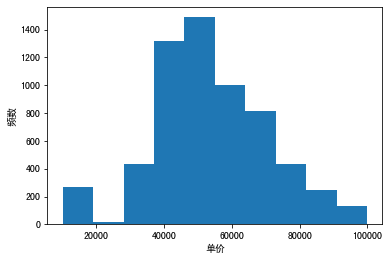#### seaborn

sns.distplot(df['单价'])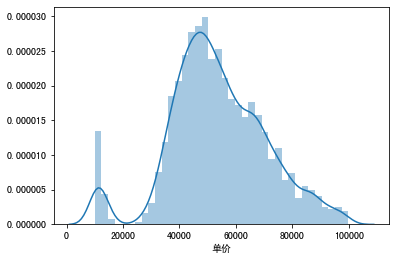### 绘制箱型图：深圳各区的房价分布、不同楼层的房价分布，设置箱型图的各项参数

sns.boxplot(data=df,y='单价',x='区');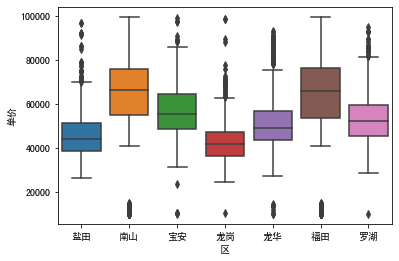sns.boxplot(data=df,y='单价',x='楼层');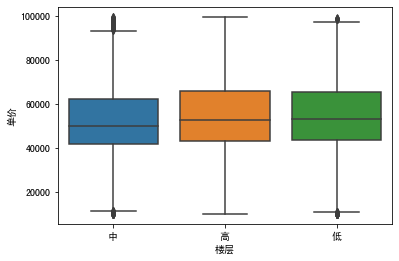### 绘制热力矩阵，查看不同变量之间的相关性强弱

sns.heatmap(df.corr())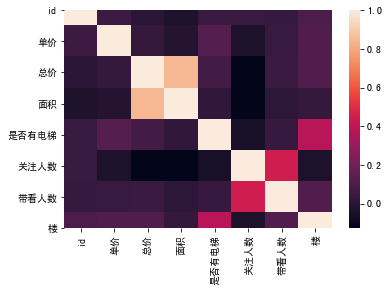### 尝试绘制 jointplot 及 pairplot

sns.jointplot(data=df,y='单价',x='面积');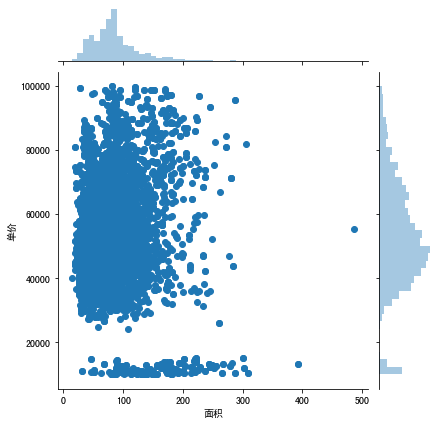sns.pairplot(df);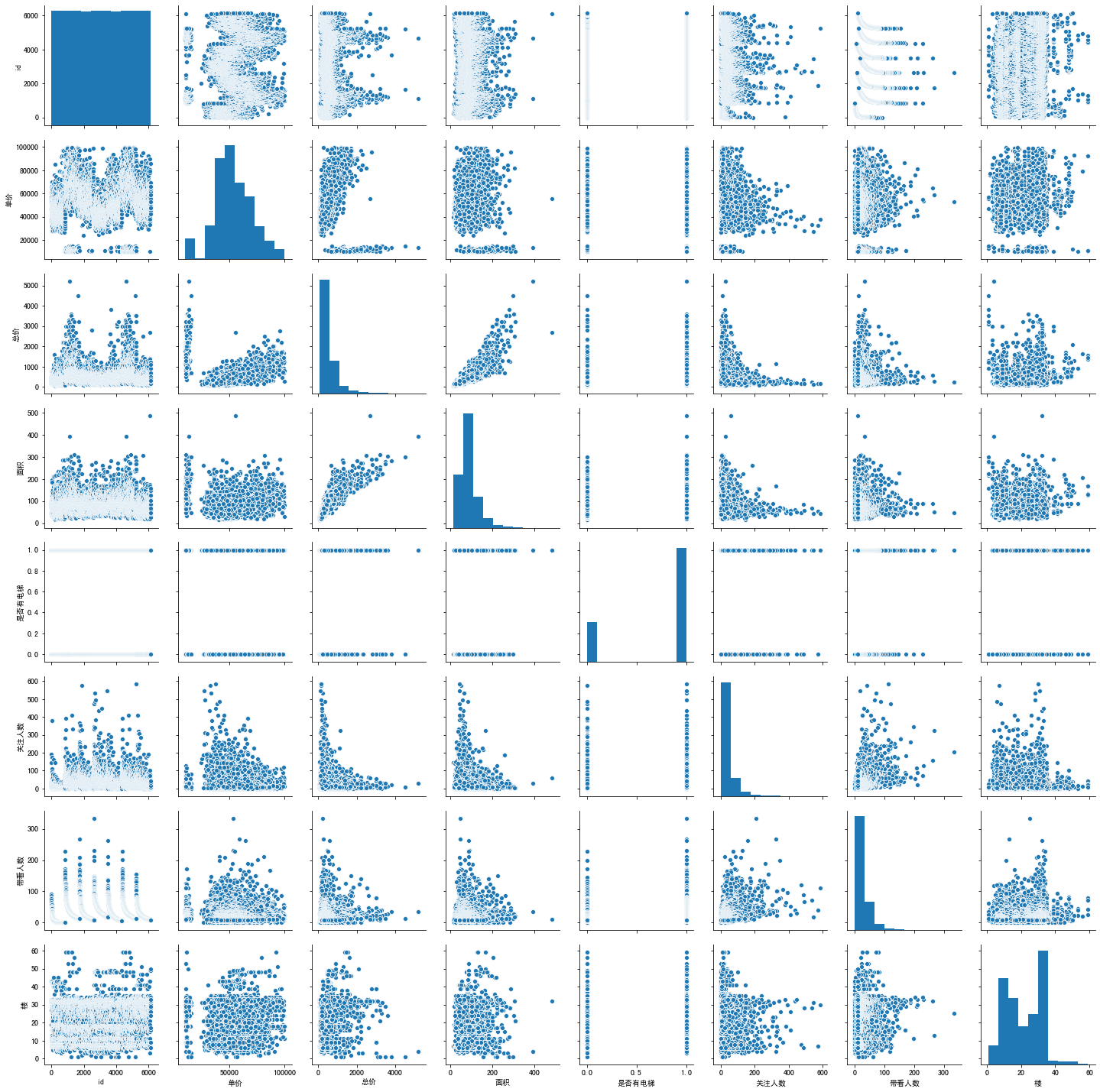### 绘制累计频率直方图：深圳单价分布

sns.distplot(df['单价'])### 通过可视化方法探索人们最关注什么户型的房子

sns.boxplot(data=df,y='关注人数',x='户型');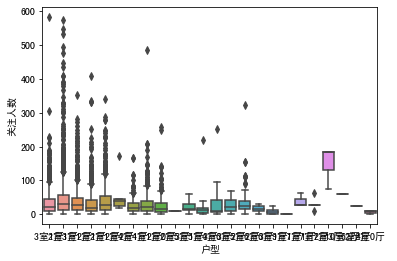### 自定义不同的价格区间绘制深圳单价在定义的价格区间内分布的直方图

plt.rcParams['font.sans-serif']=['SimHei']
plt.hist(df['单价'],range=(40000,60000))
plt.xlabel('单价')
plt.ylabel('频数')
plt.show()